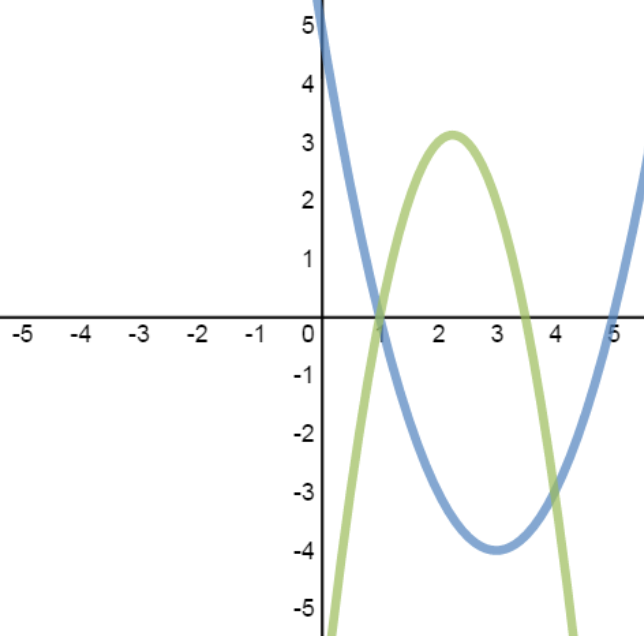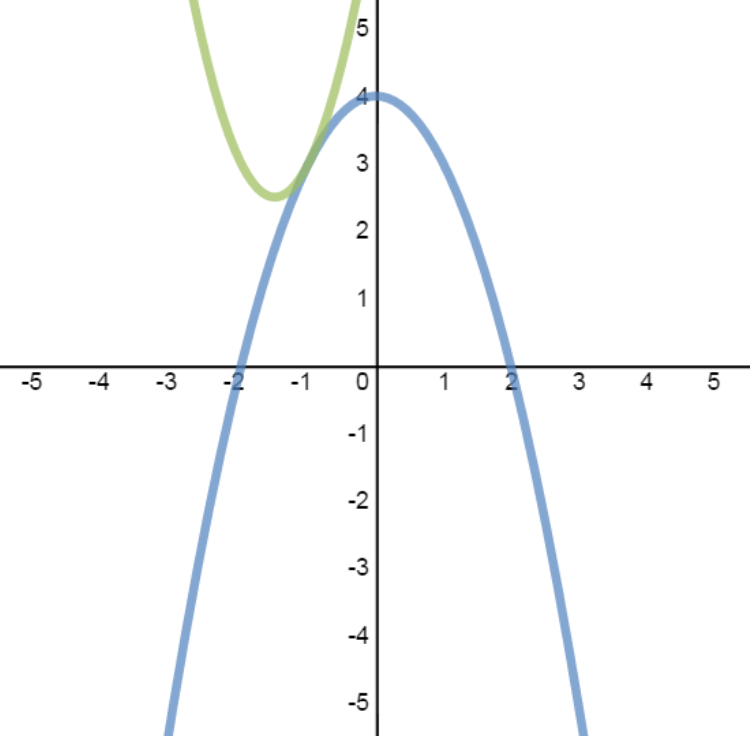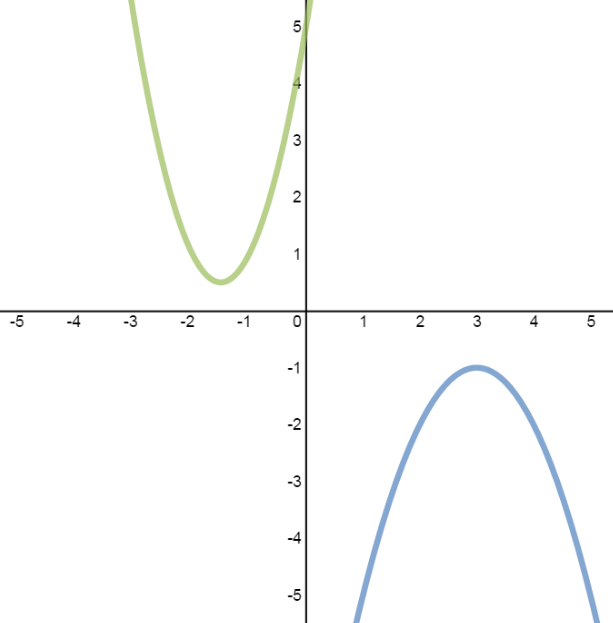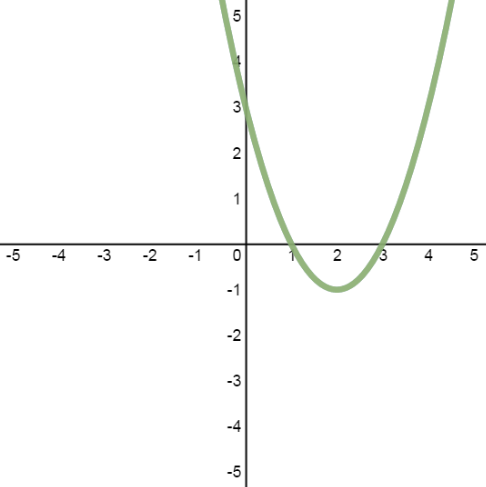The solutions to a system of equations are the points of intersection of the lines. For a system with two quadratic equations, there are 4 cases to consider: 2 solutions, 1 solution, no solutions, and infinite solutions.

#### Lessons

• Introduction
• The solutions to a system of equations are the points of intersection of the graphs.
• For a system consisting of two quadratic equations:
quadratic equation: $y = a{x^2} + bx + c$
quadratic equation: $y = d{x^2} + ex + f$
There are 4 cases to consider:
case 1: 2 solutions case 2: 1 solution case 3: no solutions case 4: infinite solutions• 1.
Case 1: System with 2 Solutions
Solve the system, then verify the solutions graphically:
$y = {x^2} - 6x + 5$
$y = - 2{x^2} + 9x - 7$

• 2.
Case 2: System with 1 Solution
Solve the system, then verify the solutions graphically:
$y = 2{x^2} + 6x + 7$
$y = - {x^2} + 4$

• 3.
Case 3: System with No Solutions
Solve the system, then verify the solutions graphically:
$y = - {x^2} + 6x - 10$
$y = 2{x^2} + 6x + 5$

• 4.
Case 4: System with Infinite Solutions
Solve the system, then verify the solutions graphically:
${x^2} - 4x - y + 3 = 0$
$5y - 5{x^2} + 20x - 15 = 0$